Hans Walser, , 

Pythagorean Triangles

We give a visualization of the usual parameterization of the Pythagorean triangles.

# 2     Parameterization of the Pythagorean triangles

Consider integers m > n > 0, m and n relatively prime and with opposite parity.

Set a = m2n2, b = 2mn, c = m2 + n2. The numbers a, b, and c are a primitive Pythagorean triple and form a primitive Pythagorean triangle. Any primitive Pythagorean triple is given by such a pair m and n (Dickson 1920), (Dickson 1966)) und (Sierpiński 1962).

Table 1 gives the first examples.

 m n a b c 2 1 3 4 5 3 2 5 12 13 4 1 15 8 17 4 3 7 24 25 5 2 21 20 29 5 4 9 40 41

Tab. 1: Pythagorean triples

# 3     Visualization

The following figures refer to the cases m = 2, n = 1 (left), and m = 5, n = 2 (right), but the corresponding figures work for any Pythagorean triangle.

We consider not only the Pythagorean triangle itself (yellow), but also the right triangle with legs m and n (red).

Figure 1 gives the basic structure according to (Foster 2016).Fig. 1: Pythagorean yellow triangle and red triangle

And now a gallery of pictures. The proofs follow from the definitions of a, b, and c. For incircle and excircles compare (Baptist 1982).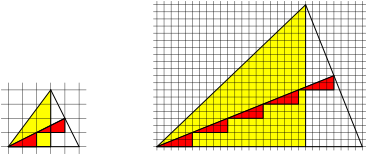Fig. 2: m red triangles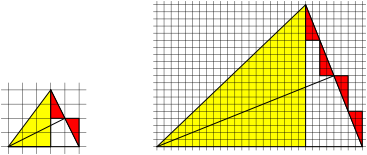Fig. 3: To times n red trianglesFig. 4: m red triangles plus n red trianglesFig 5: m red triangles minus n red trianglesFig. 6: m red triangles plus n red triangles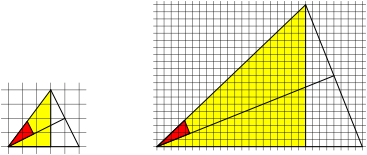Fig. 7: Rotated red triangleFig. 8: Rotated and stretchedFig. 9: m rotated plus n rotated red triangles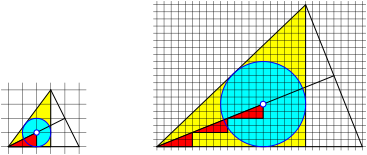Fig. 10: Incircle: (mn) red triangles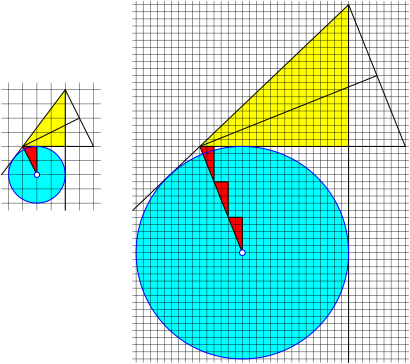Fig. 11: Excircle: (mn) red trianglesFig. 12: Excircle: (m + n) red triangles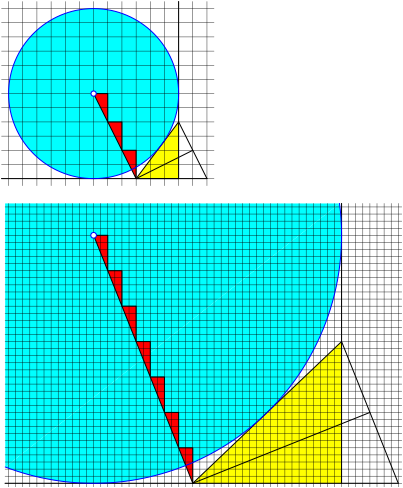Fig. 13: Excircle: (m + n) red triangles

References

Baptist, Peter (1982): Inkreisradius und pythagoreische Zahlentripel. Praxis der Mathematik, 24, 161-164.

Dickson, Leonard Eugene (1920): History of the Theory of Numbers, II. Diophantine Analysis. Washington: Carnegie Institution.

Dickson, Leonard Eugene (1966): History of the Theory of Numbers; vol II. New York: Chelsea.

Foster, Colin (2016): Proof Without Words: Integer Right Triangle Hypotenuses Without Pythagoras. The College Mathematics Journal. Vol. 47, No. 2, March 2016, 101.

Sierpiński, Wacław (1962): Pythagorean Triangles. Trans. A. Sharma. Yeshiva Univ., New York, 1962. Reprinted by Dover, Minneola, NY, 2003.